Tamilnadu State Board New Syllabus Samacheer Kalvi 12th Business Maths Guide Pdf Chapter 10 Operations Research Ex 10.1 Text Book Back Questions and Answers, Notes.

## Tamilnadu Samacheer Kalvi 12th Business Maths Solutions Chapter 10 Operations Research Ex 10.1

Question 1.
What is transportation problem?
Solution:
The objective of transportation problem is to determine the amount to be transported from each origin to each destinations such that the total transportation cost is minimized.Question 2.
Write mathematical form of transportation problem.
Solution:
Let there be m origins and n destinations. Let the amount of supply at th i th origin is ai. Let the demand at j th destination is bj.
The cost of transporting one unit of an item from origin i to destination j is Cij and is known for all combination (i,j). Quantity transported from origin i to destination j be xij.

The objective is to determine the quantity xij to be transported over all routes (i,j) so as to minimize the total transportation cost. The supply limits at the origins and the demand requirements at the destinations must be satisfied.
The above transportation problem can be written in the following tabular form: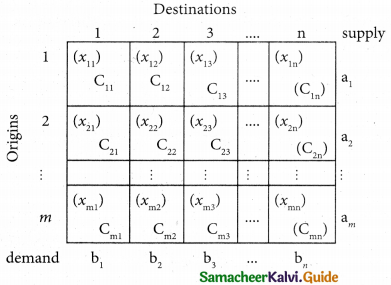Now the linear programming model representing the transportation problem is given by
The objective function is Minimize Z = $$\sum_{\mathbf{i}=\mathbf{1}}^{\mathbf{m}}$$, $$\sum_{\mathbf{j}=\mathbf{1}}^{\mathbf{n}}$$ cij xij
Subject to the constraints
$$\sum_{\mathbf{j}=\mathbf{1}}^{\mathbf{n}}$$ = xij = ai, i = 1, 2 …….. m (supply constraints)
$$\sum_{\mathbf{i}=\mathbf{1}}^{\mathbf{m}}$$ = xij = bj, i = 1, 2 …….. n (demand constraints)
xij ≥ 0 for all i, j (non- negative restrictions)

Question 3.
What is feasible solution and non degenerate solution in transportation problem?
Solution:
Feasible Solution:
A feasible solution to a transportation problem is a set of non-negative values xij (i = 1, 2, … m, j = 1, 2, … n) that satisfies the constraints.

Non degenerate basic feasible solution:
If a basic feasible solution to a transportation problem contains exactly m + n – 1 allocations in independent positions, it is called a Non degenerate basic feasible solution. Here m is the number of rows and n is the number of columns in a transportation problem.

Question 4.
What do you mean by balanced transportation problem?
Solution:
In a transportation problem if the total supply equals the total demand (Σai = Σbj) then it is said to be balanced transportation problem.Question 5.
Find an intial basic feasible solution of the following problem using north west corner rule.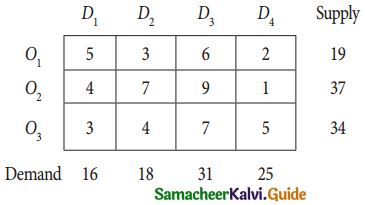Solution:
Here total Supply 19 + 37 + 34 = 90
Total demand = 16 + 18 + 31 + 25 = 90
(i.e) Total supply = Total demand
∴ The given problem is balanced transportation problem
∴ we can final an initial basic feasible solution to due given problem.

From the above table we can choose the cell in the North west corner. Here the cell is (Q1, D1). Allocate as much as possible in this cell so that either the capacity of first row is exhausted or the destination requirement of the first column’s exhausted.
(i.e) x11 = min (19, 16) = 16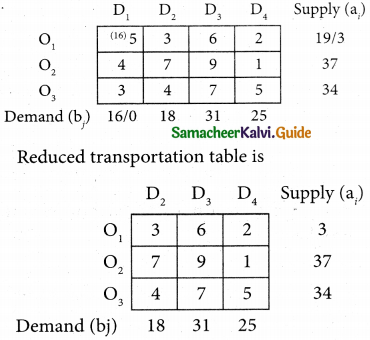Now the cell in the north west corner is (O1, D2) Allocate as much as possible in the first cell so that either the capacity of second row is exhausted or the destination requirement of the first column is exhausted.
(i.e) x12 = min (3, 18) = 3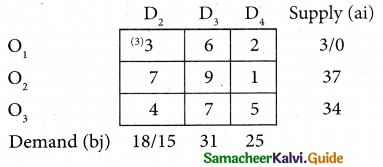Reduced transportation table is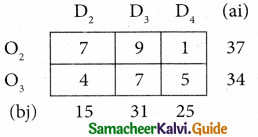Now the cell in the north west corner is (O2, D2)
x22 = min (37, 15) = 15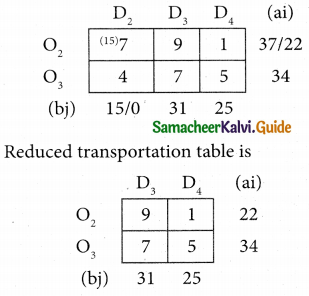Now the cell in the north west corner is (O2, D3)
x23 = min (22, 31) = 9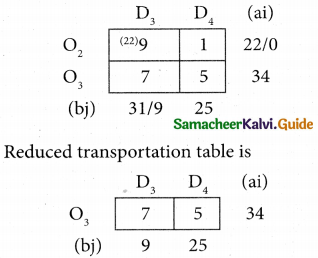Now the cell in the north west corner is (O3, D3)
x33 = min (34, 9) = 9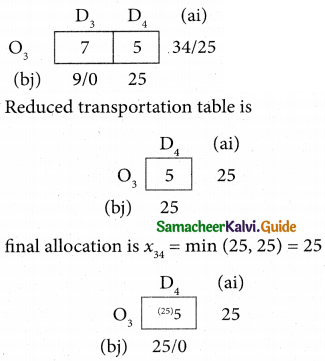Thus we have the following allocations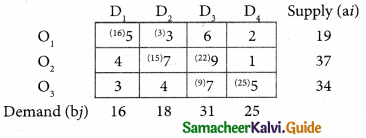Transportation schedule:
O1 → D1, O1, → D2, O2 → D2; O2 → D3 O3 → D3; O3 → D4
= (16 × 5) + (3 × 3) + (15 × 7) + (22 × 9) + (9 × 7) + (25 × 5)
= 80 + 9 + 105 + 198 + 63 + 125
= 580Question 6.
Determine an intial basic feasible solution of the following transportation problem by north west corner method.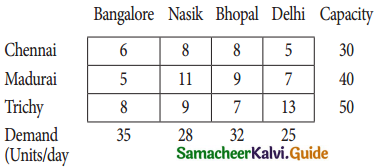Solution:
Here total capacity (ai) = 30 + 40 + 50 = 120
Total demand (bj) = 35 + 28 + 32 + 25 = 120
(i.e) Total capacity = Total demand
∴ The given problem is balanced transportation.
∴ We can find an initial basic feasible solution to the given problem.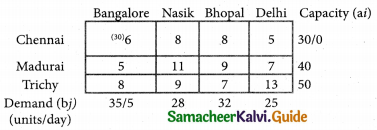From the above table we can choose the cell in the North west corner. Here the cell is (chennai, Bangalore)
x11 = min (30, 35) = 30
Reduced transportation table is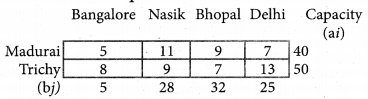Now the cell in the North west corner is (Madurai, Bangalore)
x21 = min(40, 5) = 5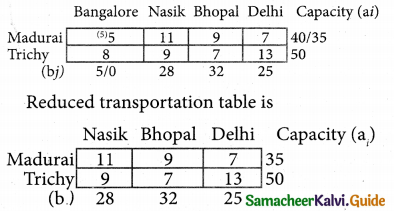From the above table we can choose the cell in the North west corner. Here the cell is (Nasik, Madurai)
x22 = min (35, 28) = 28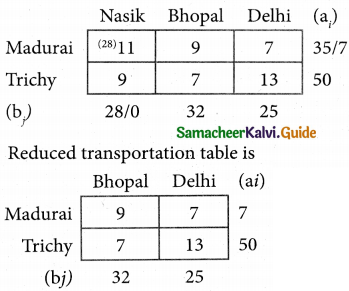From the above table we can choose the cell in the North west corner. Here the cell is (Bhopal, Madurai)
x22 = min (7, 32) = 7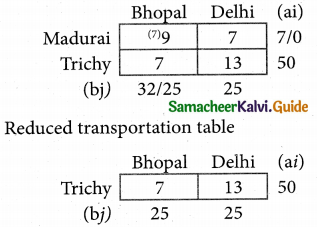From the above table we can choose the cell in the North west corner. Here the cell is (Trichy, Bhopal)
x33 = min (50, 25) = 25
Reduced transportation table is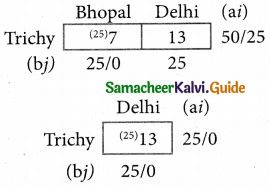x34 = min (25, 25) = 25
Thus we have the following allocations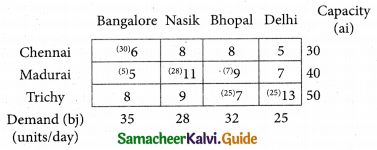Transportation Schedule:
Chennai → Bangalore; Madurai → Bangalore;
Trichy → Bhopal; Trichy → Delhi
The total transportation cost =
(30 × 6) + (5 × 5) + (28 × 11) + (7 × 9) + (25 × 7) + (25 × 13)
= 180 + 25 + 308 + 63 + 175 + 325
= 1076Question 7.
Obtain an initial basic feasible solution to the following transportation problem by using least-cost method.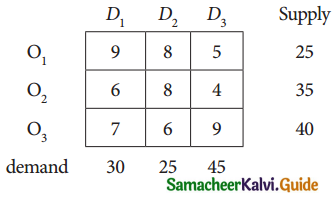Solution:
The given transportation table is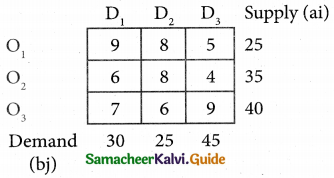Total supply = 25 + 35 + 40 = 100
Total demand = 30 + 25 + 45 = 100
(i.e) Total supply = Total demand
∴ The given problem is a balanced transportation problem.
Hence there exists a feasible solution to the given problem.
The least cos is 4 corresponds to the cell (O2, D3)
Allocate min (35, 45) = 35 units to this cell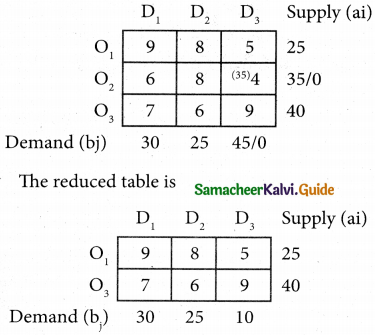The least cost corresponds to the cell (O1, D3)
Allocate min (25, 10) = 10 units to this cell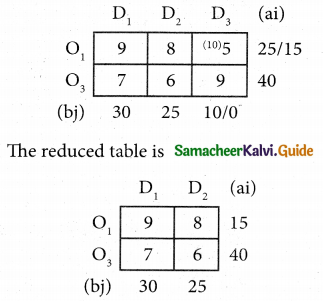The least cost is 6 corresponds to the cell (O3, D2)
Allocate min (40, 25) = 25 units to this cell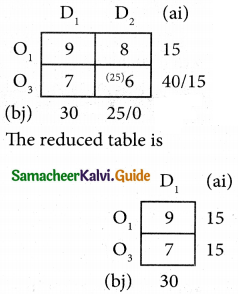The least cost is 7 corresponds to the cell (O3, D1)
Allocate min (15, 30) = 15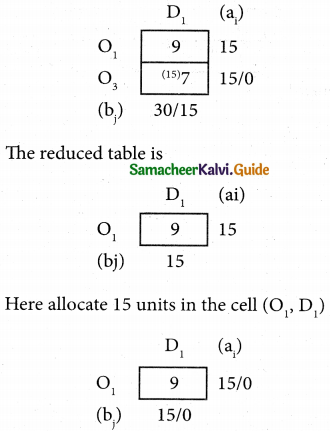Thus we have the following allocations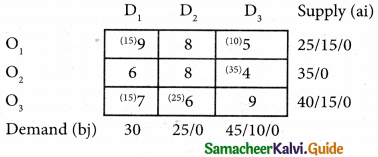Transportation Schedule:
O1 → D1; O1, → D3; O2 → D3; O3 → DI; O3 → D2
Total Transportation cost
= (15 × 9) + (10 × 5) + (35 × 4) + (15 × 7) + (25 × 6)
= 135 + 50 + 140 + 105 + 150
= 580Question 8.
Explain vogel’s approximation method by obtaining initial feasible solution of the following transportation problem.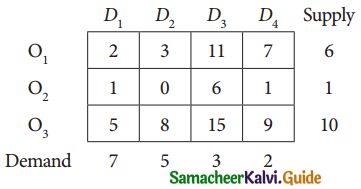Solution:
Here Σ ai = 6 + 1 + 10 = 17
Σ bj = 7 + 5 + 3 + 2 = 17
Σ ai = Σ bj
(i.e) Total supply = Total Demand
∴ The given problem is balanced transportation problem
Hence there exists a feasible solution to the given problem.
First let us find the difference (penalty) between the first two smallest costs in each row and column and write them in brackets against the respective rows and columns.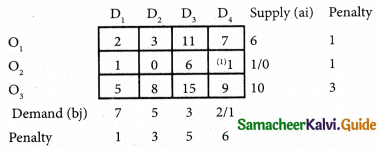Choose the largest difference. Here the largest difference is 6 which corresponds to column D4
In this column choose the least cost. Here the least cost corresponds to (O2, D4)
Allocate min (1, 2) = 1 unit to this cell the reduced transportation table is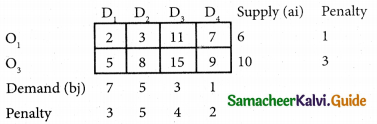choose the largest difference 5 which corresponds to column D2. Here the least cost corresponds to (O1, D2).
Allocate min (6, 5) = 5 units in this cell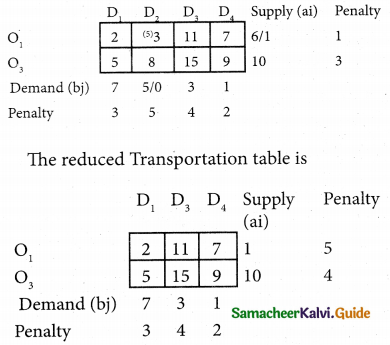Choose the largest difference 5 which corresponds to row O1. Here the least cost corresponds to (O1, D1)
Allocate min (1, 7) = 1 unit in this cell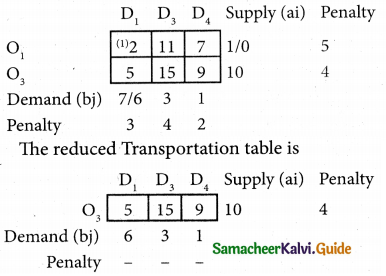Choose the largest difference 4 which corresponds to row O3. Here least cost corresponds to (10, 6) = 6 units in this cell.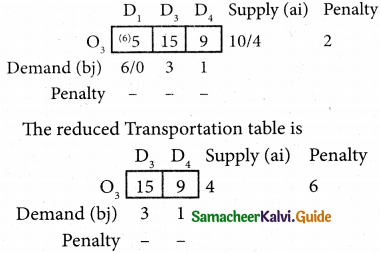Choose the largest difference 6 which corresponds to row O3. Here the least cost corresponds to (O3, D4).
Allocate min (4, 1) = 1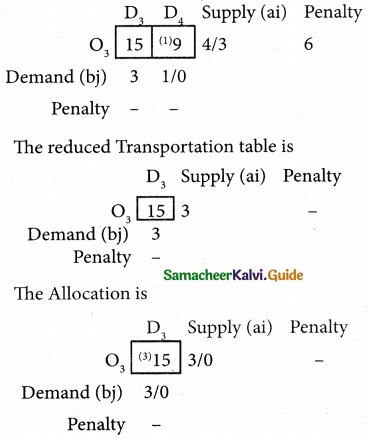Thus we have the following allocations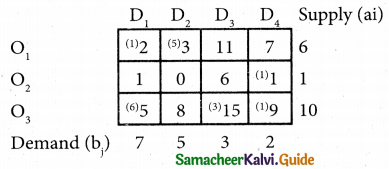Transportation schedule
O1 → D1; O1 → D2; O2 → D4;
O3 → D1; O3 → D3; O3 → D4
Total transportation cost:
= (1 × 2) + (5 × 3) + (1 × 1) + (6 × 5) + (3 × 15) + (1 × 9)
= 2 + 15 + 1 + 30 + 45 + 9
= 102Question 9.
Consider the following transportation problem.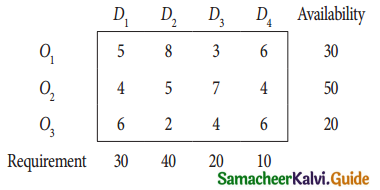Determine initital basic feasible solution by VAM
Solution:
Given Transportation problem is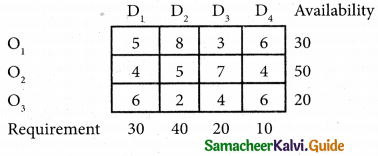Here Σ ai = Σ bj = 100
∴ The given problem is balanced transportation problem.
Hence there exists a feasible solution to the given problem.
First let us find the difference (penalty) between the first two smallest costs in each row and column and write them in brackets against the respective rows and columns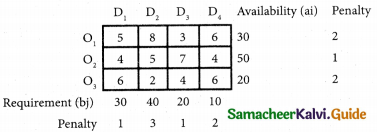Choose the largest difference. Here the difference is 3 which corresponds to D2
In this column choose the least cost. Here the least cos corresponds to (O3, D2)
Allocate min (20, 40) = 20 units to this cell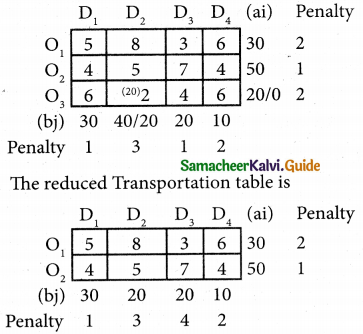Choose the largest difference is 4 which corresponds to column D3. In this column choose the least cost. Here the least cost corresponds to (O1, D3).
Allocate min (30, 20) = 20 units to this cell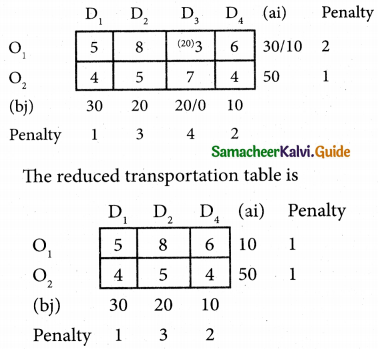Choose the largest different is 3 which corresponds to column D2. In this column choose the least cost. Here the least cost corresponds to (O2, D2)
Allocate min (50, 20) = 20 units to this cell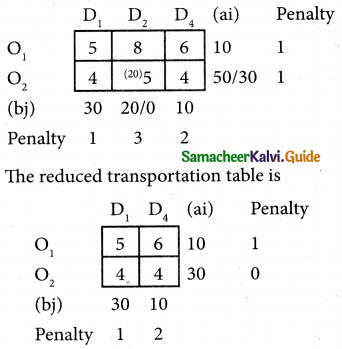Choose the largest difference is 2 which corresponds to column D4. In this column choose the least cost. Here the least cost corresponds to (O2, D4).
Allocate min (30, 10) = 10 units to this cell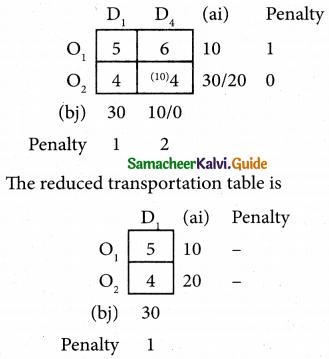Allocate min (20, 30) = 20 units to this cell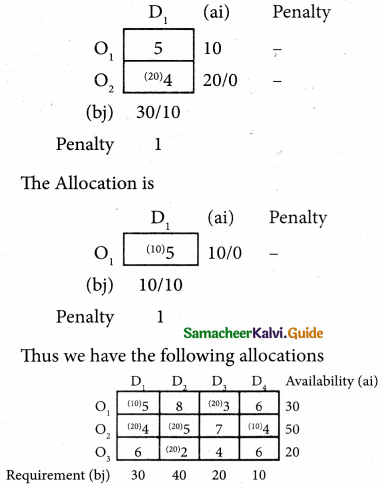Here
x11 = 10
x13 = 20
x21 = 20
x22 = 20
x24 = 10
x32 = 20
Transportation schedule
O1 → D1; O1 → D3; O2 → D1;
O2 → D2; O2 → D4; O3 → D2
The transportation cost
= (10 × 5) + (20 × 3) + (20 × 4) + (20 × 5) + (10 × 4) + (20 × 2)
= 50 + 60 + 80 + 100 + 40 + 40
= 370Question 10.
Determine basic feasible solution to the following transportation problem using North west Corner rule.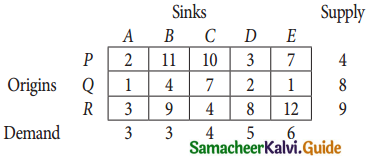Solution:
Here total supply = 4 + 8 + 9 = 21
Total demand = 3 + 3 + 4 + 5+ 6 = 21
(i.e) Total supply = Total demand
∴ The given problem is balanced transportation problem.
∴ we can find an initial basic feasible solution to the given problem.
From the above table we can choose the cell in the North west corner. Here the cell is (P, A)
Allocate as much as possible in this cell so that either the capacity of first row is exhausted or the destination requirement of the first column is exhausted.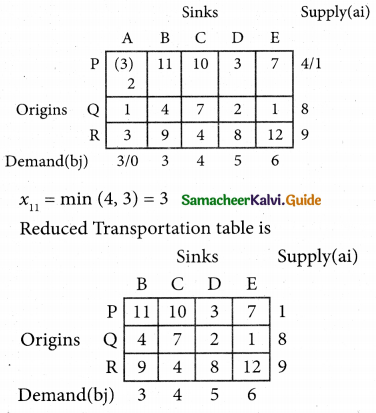Form the above table we can choose the cell in North west corner. Here the cell is (P,B)
x = min (1, 3) = 1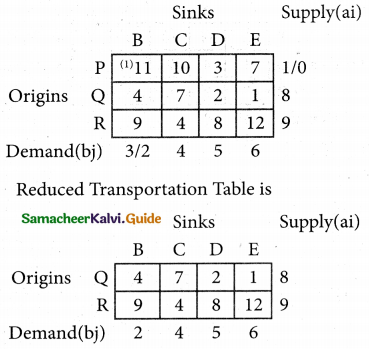From the above table we can choose the cell in north west corner. Here the cell is (Q, B)
x = min (2, 8) = 2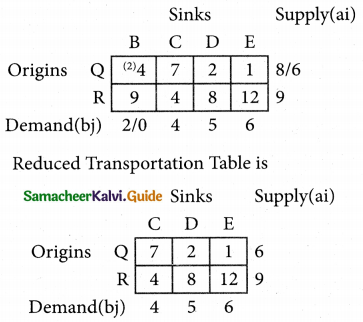From the above table, we can choose the cell in North west corner. Here the cell is (Q, C)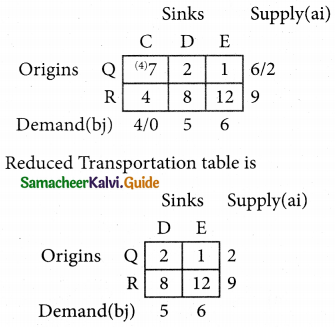From the above table, we can choose the cell in North west corner. Here the cell is (Q, D)
x = min (2, 5) = 2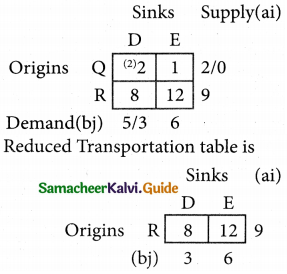From the above table, we can choose the cell in North west corner. Here the cell is (R, D)
x = min (9, 3) = 3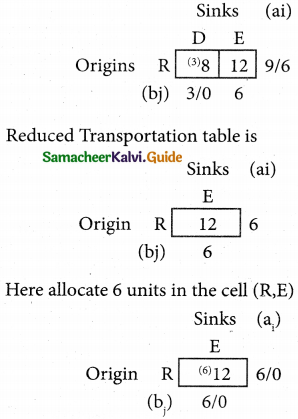Thus we have the following table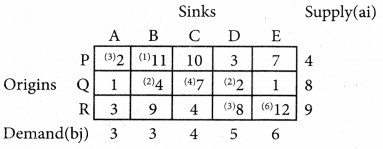Transportation Schedule:
P → A; P → B; Q → B; Q → C; Q → D R → D; R → E
Total transportation cost:
= (3 × 2) + (1 × 11) + (2 × 4) + (4 × 7) + (2 × 2) + (3 × 8) + (6 × 2)
= 6 + 11 + 8 + 28 + 4 + 24 + 72
= 153Question 11.
Find the initial basic feasible solution of the following transportation problem: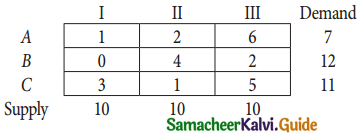Using
(i) North West corner tule
(ii) Least Cost method
(iii) Vogel’s approximation method
Solution:
(i) North west corner rule:
Here the total supply = 10 + 10 + 10 = 30
Total demand = 7 + 12 + 11 = 30
(i.e) Total supply = Total demand
The given problem is balanced transportation problem.
∴ we can find an initial basic feasible solution to the given problem.
From the above table we can choose the cell in the North west corner. Here the cell is (A, I)
x11 = min (7, 10) = 7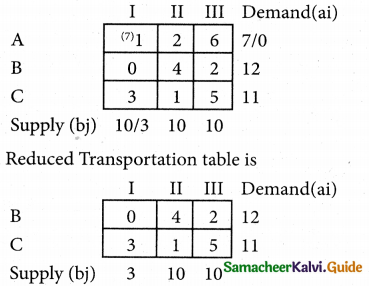From the above table we can choose the cell in the north west corner. Here the cell is (B, I)
x = min (3, 12) = 3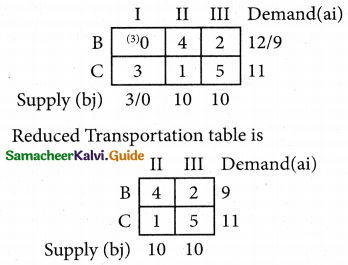From the above table we can choose the cell in the North west corner. Here the cell is (B, II)
x = min (9, 10) = 9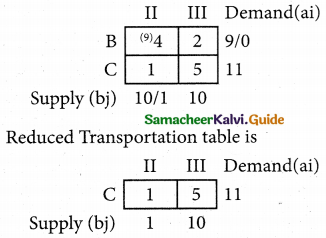Here the cell in the North west corner is (C, II)
x = min (11, 1) = 1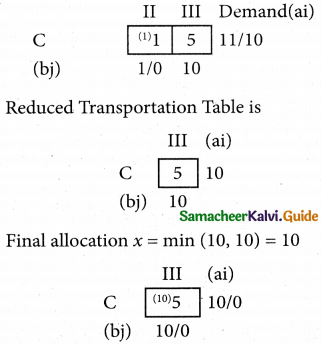Thus we have the following allocations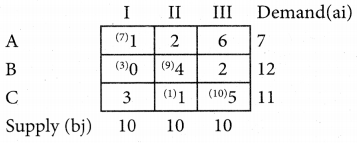Transportation schedule:
A → I; B → I; B → II; C → II; C → III
Total transportation cost:
= (7 × 1) + (3 × 0) + (9 × 4) + (1 × 1) + (10 × 5)
= 7 + 0 + 36 + 1 + 50
= 94(ii) Least cost method:
The given transportation table is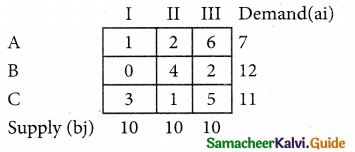Here Total supply = Total demand = 30
∴ The given problem is a balanced transportation problem.
Hence there exists a feasible solution to the given problem.
The least cost is 0 corresponds to the cell (B, I)
Allocate min (12, 10) = 10 units to this cell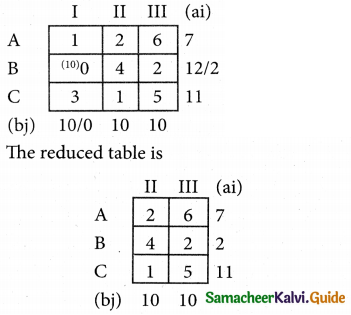The least cost 1 corresponds to the cell (C, II)
Allocate min (11, 10) = 10 units to this cell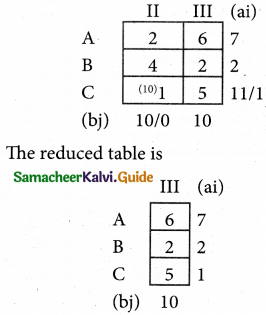Here the least cost 2 corresponds to the cell (B, III)
Allocate min (2, 10) = 2 units to this cell.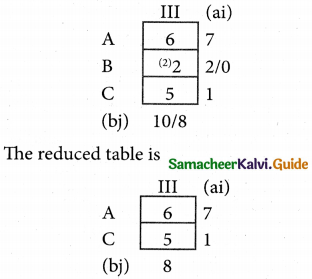Here the least cost is 5 corresponds to the cell (C, III)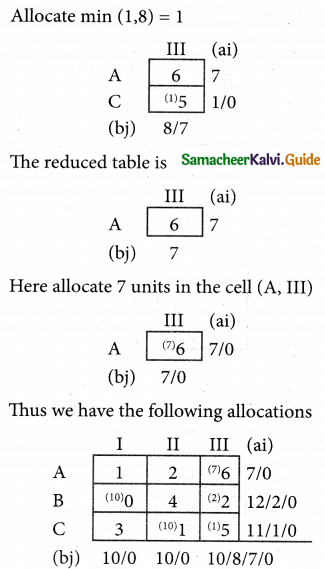Transportation schedule:
A → III; B → I; B → III; C → II; C → III
Total transportation cost:
= (7 × 6) + (10 × 10) + (2 × 2) + (10 × 1) + (1 × 5)
= 42 + 0 + 4 + 10 + 5
= 61

(iii) Vogel’s approximation method:
Here Σ ai = Σ bj = 30
(i.e) Total supply = Total demand
∴ This given problem is balanced transportation problem.
Hence there exists a feasible solution to the given problem.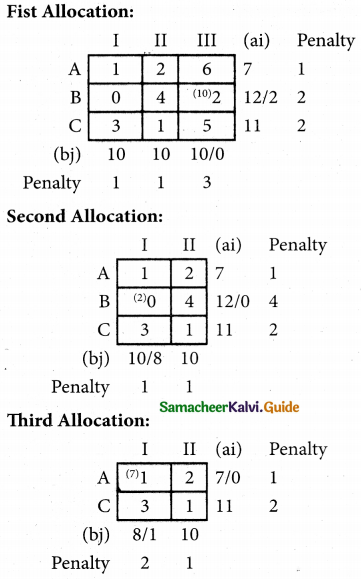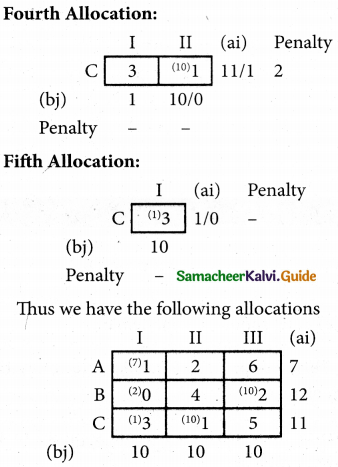A → I; B → I; B → III; C → I; C → II
Total transportation cost:
= (7 × 1) + (2 × 0) + (10 × 2) + (1 × 3) + (10 × 1)
= 7 + 0 + 20 + 3 + 10
= 40Question 12.
Obtain an initial basic feasible solution to the following transportation problem by north west corner method.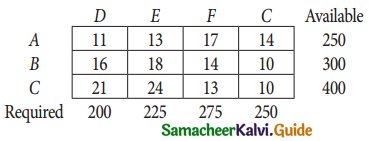Solution:
Here the total available = 250 + 300 + 400 = 950
Total Required = 200 + 225 + 275 + 250 = 950
(i.e) Total Available = total required
∴ The given problem is balanced transportation problem.
we can find an initial basic feasible solution to the given problem.
From the above table, we can choose the cell in the North west corner. Here the cell is (A, D).
x11 = min (250, 200) = 200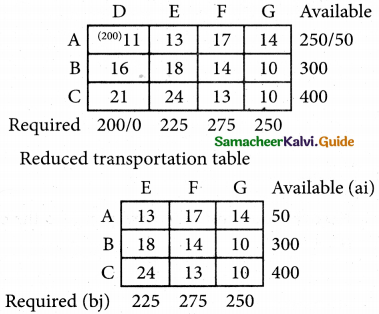From the above table we can choose the cell in North west corner. Here the cell is (A, E)
x = min (50, 225) = 50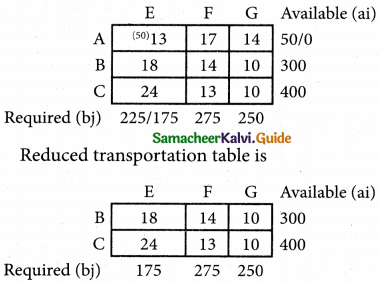From the above table, the north west corner cell is (B, E)
x = min (300, 175) = 175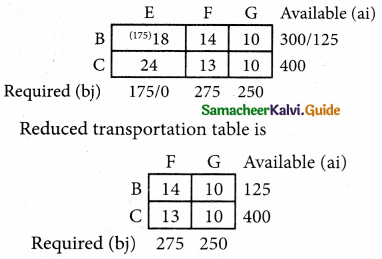From the above table, the north west corner cell is (B, F)
x = min (125, 275) = 125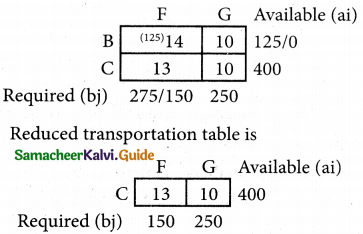Here the north west corner cell is (C, F)
x = min (400, 150) = 150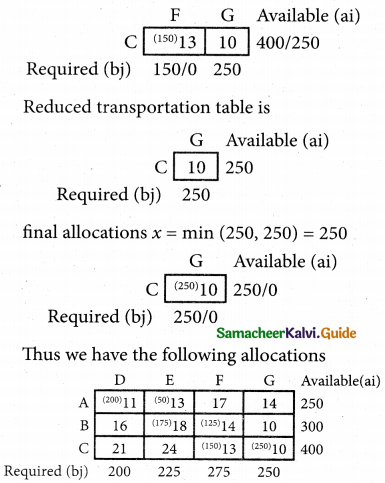Transportation schedule:
A → D; A → E; B → E; B → F; C → G
Total Transportation cost:
= (200 × 11) + (50 × 13) + (175 × 18) + (125 × 14) + (150 × 13) + (250 × 10)
= 2200 + 650 + 3150 + 1750 + 1950 + 2500
= 12,200